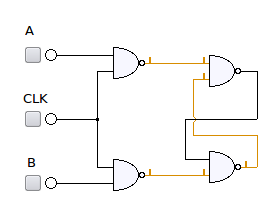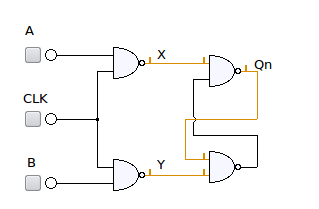# GATE-2012 ECE Q6 (digital)Question 6 on digital circuit from GATE (Graduate Aptitude Test in Engineering) 2012 Electronics and Communication Engineering paper.

## Q6. Consider the given circuitIn this circuit, the race around

(A) does not occur

(B) occurs when CLK=0

(C) occurs when CLK=1 and A=B=1

(D) occurs when CLK=1 and A=B=0

## Solution

Looking up the definition of Race Condition from Wiki :

“A race condition or race hazard is a type of flaw in an electronic or software system where the output is dependent on the sequence or timing of other uncontrollable events. The term originates with the idea of two signals racing each other to influence the output first.

A typical example of a race condition may occur in a system of logic gates, where inputs vary. If a particular output depends on the state of the inputs, it may only be defined for steady-state signals. As the inputs change state, a small delay will occur before the output changes, due to the physical nature of the electronic system. For a brief period, the output may change to an unwanted state before settling back to the designed state.”

Using the truth table of NAND gates, we know that

,

, irrespective of the values of .

The circuit shown in the figure is a gated SR latch circuit using NAND gates [Ref: 2, 3].Figure : Gated SR latch using NAND gates

The state transition behavior is as follows :

Table : State transition table for gated SR-latch using NAND gates

## Update (11th Oct 2012):

Given that there were some clarification raised by Mr. Raghav in the comments section, let us try to review the answers

 Choices Remark (A) does not occur Clearly, this is not true. The race around can occur when the input condition transitions from {X=Y=0} to {X=Y=1}.The {X=Y=0} is caused when {CLK=A=B=1}.The {X=Y=1} can be caused by either {CLK=0, A=x, B=x} inputs OR {CLK=1, A=B=0} input condition. (B) occurs when CLK=0 This is partially true.If the previous input condition was {CLK=A=B=1}, then changing to CLK=0 can cause race aroundNote : On power up, if CLK=0 then the system can go and settle into an indeterminate state. (C) occurs when CLK=1 and A=B=1 This is partially true. If the next input condition is either {CLK=0} or {CLK=1, A=B=0}, can cause race around (D) occurs when CLK=1 and A=B=0 This is partially true. If the previous input condition was {CLK=1, A=B=1}, then changing input to {CLK=1, A=B=0} can cause race around.

Based on the above, the lawyer in me feels that choices (B), (C), (D)  are all partially true. However as an engineer, the common factor for the cause of race around condition is {CLK=1, A=B=1}.

### Given so, the right choice is :  (C) i.e. race condition can occur when CLK=1 and A=B=1 (with high probability) 😉

Note: Am looking for an easy to read text book which explains in transistor level the logic gates. Please drop a comment or send me an email.

## References

 GATE Examination Question Papers [Previous Years] from Indian Institute of Technology, Madras http://gate.iitm.ac.in/gateqps/2012/ec.pdf

 SE 271 — Introduction to Digital Systems Supplementary Reading Some Basic Memory Elements http://ecse.bd.psu.edu/cse271/memelem.pdf

 Digital Electronics http://ptuece.loremate.com/die/node/11

 Sequential Circuits http://www.elex.dauniv.ac.in/Kirti_Elex/PDF/Sequential_Circuit_1.pdf

 SR Flip-Flop http://www.electronics-tutorials.ws/sequential/seq_1.html

## 6 thoughts on “GATE-2012 ECE Q6 (digital)”

1.Manu says:

A race condition is simply when the output levels depend on the two timing paths that are not dependant on each other. Now when the clock goes down to 0, when A and B were 1, it is not possible to predict what the value of Qn or Qn’ would be. Therefore there is a race condition when CLK = 0.

1.Krishna Sankar says:

@Manu: Thanks for the clarification. Yes, I agree to your observation.

2.Raghava G D says:

I think the reason you have given for the answer to be C, is for a “race condition” . But the question i guess is about “race around” which is the state wherein the output keeps toggling if the clock pulse width is more than the delay through the gates.

In the above circuit, when Clk = A = B = 1, Qn and Qn’ be equal to 1.
Now when Clk switches to 0, if the delays through the nand gates are assumed constant ( which in probability is not true!!) then the output keeps toggling between 0 and 1.

But assuming the delays through the gates cannot be assumed constant, the race around condition cannot occur!! ..So i would go for option A.

1.Krishna Sankar says:

@Raghava: Thanks for the detailed reply.
I too had a similar doubt, but few points which made me think otherwise:
a) Race condition and race around condition are kind of similar. In the former, the output glitches due to the delay in the inputs. In the race around case, there is dependency on the outputs (like IIR filter case) and cause glitches.
b) Let me try to draw in the CMOS level circuit and try to rationalize why the output can not be determined in the CLK=A=B=1 state.

1.Raghava G D says:

I guess in race condition, there need not be a glitch in output. It is a condition wherein the steady state output is dependent on the delay through the gates.

For ex in SR flip flop, when both S and R is equal to 1 both Q and Q’ will be equal to 0. So under this condition when S and R both change to 0 simultaneously the outputs Q and Q’ will be dependent on the delay through the nor gates, i.e S and R will race with each other to determine the state.

Even in the above circuit, it is not that output cannot be determined when Clk = A = B = 1. The output can surely be determined and will be equal to 1 on both Q and Q’. But just that it is a prohibited state since logically Q’ will not be complement of Q and the next state when both the inputs change to 0 simultaneously cannot be determined, because as explained above inputs, race to change the output and will depend on the delay through the gates.

i don’t know if i am right, i have just written what i have understood, kindly clarify.

1.Krishna Sankar says:

@Raghava: Yes, you are right. When CLK=A=B=1 condition is followed up by CLK=0 OR {CLK=1,A=B=0}, race around can occur. Triggered by your comment, felt the need for updating the blog post. Please checkout the Update (11th Oct 2012) section in the post and revert.

Thanks again! 🙂